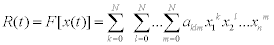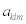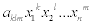Reach Us+44-7482876202
Non-Linear Series Inversion Method for Forecasting Canadian GDP Growth | OMICS International
Business and Economics Journal
All submissions of the EM system will be redirected to Online Manuscript Submission System. Authors are requested to submit articles directly to Online Manuscript Submission System of respective journal.

# Non-Linear Series Inversion Method for Forecasting Canadian GDP Growth

Lee T.-W.*

School for Engineering of Matter, Transport and Energy, Arizona State University, Tempe, AZ 85287-6106, USA

*Corresponding Author:
Lee TW
School for Engineering of Matter Transport
and Energy, Arizona State University
Tempe, AZ 85287-6106, USA
Tel: (480)965- 7989
E-mail: [email protected]

Received Date: June 23, 2015 Accepted Date: July 01, 2015 Published Date: July 08, 2015

Citation: Lee TW (2015) Non-Linear Series Inversion Method for Forecasting Canadian GDP Growth. Bus Eco J 6:165. doi:10.4172/2151-6219.1000165

Copyright: © 2015 Lee TW. This is an open-access article distributed under the terms of the Creative Commons Attribution License, which permits unrestricted use, distribution, and reproduction in any medium, provided the original author and source are credited.

Visit for more related articles at Business and Economics Journal

#### Abstract

We present a new method for automatically generating mathematical models of complex, non-linear processes, and apply it for tracking and predicting the Canadian GDP. This method is derived from solving complex, non-linear problems in engineering, and is found to be an efficient method for forecasting of financial and economic variables. The method involves setting up a general non-linear series involving terms of up to 3rd-order products, where the model coefficients are systematically determined by the data on the Canadian GDP along with significant economic indicators such as currency, gross demand deposits, consumer price index and various loan rates. Results show that GDP can be predicted quantitatively and qualitatively, at various prediction intervals, with longer-term predictions showing less agreement owing to divergent dynamics in the economic variables and GDP. Other complex financial and economic processes may be analyzed and predicted using this method.

#### Keywords

Inversion method forecasting; GDP growth

#### Introduction

Prediction of economic parameters such as gross domestic product (GDP) growth is important for future planning and policy-making. In particular, finding the functional relationship, or a mathematical model, between GDP growth and some key indicator variables is the goal of many economic analyses and studies . However, interaction of the variables can be quite complex and non-linear, and some key model parameters are not easily determined and highly variable in time. Therefore, several statistical and analytical methods have been attempted with varying degrees of success [2-8]. This is where a parallel exists in between the current problem and many non-linear problems in social, physical sciences and engineering: Non-linear processes are subject to unpredictable behavior, and are difficult to model. Indeed, methods such as neural network  have been applied in numerous non-linear problems in science and engineering.

In this work, we describe a new method for modeling non-linear processes, called non-linear series inversion, and demonstrate its utilities. Similar to the neural network modeling, this method has been developed to predict or model the non-linear processes in dynamical systems, such as chaotic oscillator , and flow turbulence and chemical reactions. The method is data-driven, and automatically generates the parameters in the non-linear model. It is a generalized method, which can be applied to other complex financial and economic problems. The target parameter, to be predicted, is written as a non-linear series expansion involving the relevant variables with initially undetermined coefficients. The coefficients are then determined using a reference set of data through matrix inversion. If the correct set of variables is inserted in the non-linear series in an appropriate manner, then it is found that a reliable model can be built to predict the process variables under a wide range of initial/boundary conditions. The matrix inversion for determination of the coefficients can be done through compressive sensing algorithm, although other matrix inversion method can be employed. The significance is that this approach is applicable to a wide range of non-linear flow processes, where limited knowledge of detailed interaction between variables and unknown model coefficients can preclude or hamper accurate predictions.

#### Description of the Method and Results

Complex phenomena typically involve interactions of several or many variables. Predicting the GDP growth is also expected to involve complex interactions that cannot easily be identified or quantified. For these complex, non-linear problems, a method has been developed in this laboratory that takes into account of main key variables (such as the key economic indicators) and transforms the variables as a series of non-linear terms. Then, the coefficients of the non-linear series are found by using the actual outcome or data for the processes to be predicted.

We can start by representing the percent change of the GDP in some time interval, as the outcome to be predicted. The factors that will affect this percent change are many; however, we choose the key economic indicators likely to have large impact on GDP growth/ decline. Another criterion for selecting the economic indicators is that they should readily be available, so that implementation is straightforward and timely. For study of GDP, the following parameters have been widely used, and also their data are readily available at quarterly intervals: short-term interest rate, long-term interest rate, price level (consumer price index), inflation expectation, currency+gross demand deposits. However, in many instances  these variables are combined in a form to reflect trends in the economy. The combined variables are defined as follows:

x1=M1/CPI

x2=M2/CPI

x3=(R10-R90)

x4=(R90-xp)

where M1=currency+gross demand deposits

M2=M1+non-personal notice deposits+personal savings deposits

CPI=consumer price index

R10=average yield of Government of Canada marketable bonds, 10 years and over

R90=90-day prime corporate paper rate

xp=100log10(CPI/CPI-4)

CPI-4=CPI at the previous 4th quarter

These data, along with the GDP for each quarter are readily available from Statistics Canada’s CANSIM data base, and we have used the data from 1968 up to 2011. Since the magnitude of the variables vary over a large range, the data are normalized with respect to each variable value at some reference time, say 1st quarter of 1995.

The basic non-linear series that is often used is in the following form.Here, R(t) is the percent change in Canadian GDP with respect to some reference quarter, 1, 2, 4, or 5th prior quarter. The quarter is marked with t, so that t=5 for example would be 1 year and 1 quarter from the reference quarter. The coefficients for each term in the series, is, and k, l, and m are the exponents. For some non-linear processes such as turbulence flows, this type of series worked reasonable well when the exponents were allowed to vary from 1 to 4. However, for prediction of economic processes, inclusion of exponents greater than three leads to anomalous numerical results, which indicates the degree of non-linearity (the exponents) is not very high. Thus, we only include up to cubic terms (exponent of 2) to formulate a series which consists of 32 coupled terms. If it turns out that the higher-order terms do not impact GDP, then the matrix inversion will return small coefficients for these terms. The magnitude of the coefficients in fact can indicate the dominant coupling of the parameters.

With this non-linear series and the data for Canadian GDP and other economic variables listed above, we can determine the coefficients,, through a numerical matrix inversion. For this matrix inversion, R(t) on the left-hand side of Eq.1 is the data for the percent change in Canadian GDP (from CANSIM data set), while the right-side includes theof which the products of the variables again are computed from the CANSIM data.

This way, the unknowns are the coefficients, which are obtained from matrix inversion of Equation 2. If the data are sparse, then compressive sensing algorithms are used; however, in this case there is a full set of data so that a simple matrix inversion algorithm suffices. Once the non-linear series “model” has thus been created, with the coefficients, then it can be applied for comparison with existing data and for future predictions.

First, the non-linear series coefficients are determined using the known percent change in the Canadian GDP, from 1968 to 2010. CANSIM publishes the quarterly Canadian GDP, from which the percent change in one, two, or any arbitrary number of quarters, from the current quarter, can be computed. Here, we use the entire range of the data from 1968 to 2010 to determine the coefficients. Once these coefficients have thus been determined, we can re-compute the percent change in GDP using Eq 2. In Figure 1, we compare the computed results (Eq 2), with the data for the percent change in two and five quarters. It can be seen that the many of the details of the GDP percent change are reproduced by the constructed model. The accuracy is much better for the 2-quarter predictions (predicting the GDP percent change two quarters ahead of the given quarter, based on the economic indicator variables at that quarter). The significant downturns in the Canadian economy, as represented by negative percent change, in 1982 and 1990 are quite accurate tracked. Interestingly, the downturn in 2008 is not captured, indicating that that event was unique and the correlations between the GDP and economic indicators were unprecedented. The upswing in the GDP is much better captured by the 2-quarter prediction model than the 5-quarter predictions. The larger time gap, between the current economic indicators and GDP change to be predicted after five quarters, leads to less correlation between these parameters, and therefore less accurate predictions. Nonetheless, the overall tracking of the GDP change is quite good, with many of the up- and down-swings predicted by this method.

Figure 1: Comparison of GDP data with the model results, where the non-linear series coefficients are determined using the known GDP values.

However, even though we have used the term “predictions” to describe the results in Figure 1, strictly speaking the computed results are not true predictions, since the known GDP from 1968 to 2010 were used to determine the coefficients in the non-linear series in Figure 1. A true test of the methodology would be to use only the prior data, GDP and economic indicator variables, and determine the coefficients in the non-linear series, to calculate the GDP percent change in the quarters ahead of time. This can be implemented by creating a moving window of data, where we start from some initial time and use the GDP data up to a time, say 20XX, Yth quarter and use only those data to determine the coefficients in the non-linear series, in order to predict the GDP percent change at 20XX, (Y+1)th or at some quarter ahead of 20XX, Yth quarter. Then, obviously we can use the data up to any point, say 2014, 2nd quarter, to predict the GDP percent change at 2014, 3rd or any time after. The results of these true predictions are plotted in Figures 2-4 for +1, +2 and +4 quarter predictions, respectively.

Figure 2: Comparison of GDP data with the model results, where the non-linear series coefficients are determined using only the prior GDP values. Predictions are for +1 quarter ahead of time.

Figure 3: Comparison of GDP data with the model results, where the non-linear series coefficients are determined using only the prior GDP values. Predictions are for +2 quarter ahead of time.

Figure 4: Comparison of GDP data with the model results, where the non-linear series coefficients are determined using only the prior GDP values. Predictions are for +4 quarter ahead of time.

The +1 quarter predictions shown in Figure 2 indicate that the current method is capable of tracking the up- and down-swings in GDP fairly accurately. The line represents the predicted values, while symbols are used to denote the actual data. There are some overshoots in the predictions near 2000, 2006 and particularly 2008, where the non-linear series model is not able to predict the sudden downturn, due to the real-estate collapse at that time. The latter is due to the anomalous nature of that downturn event, where economic indicators were not correlated with the actual event. The +2 and +5 quarter predictions follow the data qualitatively, but perform less well due to further spacing between the available data and the quarter to be predicted. Nonetheless, it is notable that this method is able to provide predictions that follow the actual data based on a relatively simple non- linear series inversion, without either a complex model to relate the economic variables nor the need for any arbitrary parameters to fit the model predictions with the data. The method is data-driven in that the non-linear series coefficients are evaluated using a moving window of data, and the duration of this data window is the only parameter that is used to optimize the predictions, along with of course the form of the non-linear series. As noted above, inclusion of higher-order terms led to anomalous output quantities, which indicates that the interaction of the economic variables are only weakly non-linear.

We can identify the important economic variables and their interactions, by examining the coefficients in the non-linear series, as shown in Figure 5 where the first 14 coefficients are plotted. The largest coefficients are for the i=1, 5 and 10, and these correspond to linear terms of x1=M1/CPI, x3=(R10-R90) and x4=(R90-xp), respectively. The definition of these variables has been listed in Eq 1. The next significant coefficients are i=2, 4, 7, 11 and 14, and these involve quadratic products of x1, x2, and x4. Thus, it appears that the low-order terms in the nonlinear series dominate the expression, and higher-order terms only add small modifications to the output results.

Figure 5: Coefficient values for the first 14 terms in the non-linear series, used to generate predictions shown in Figure 4.

#### Conclusion

A method for automatically generating mathematical models of complex, non-linear processes has been applied for tracking and predicting the Canadian GDP. The method uses a non-linear series involving terms of up to 3rd-order products, where the coefficients are determined by the data on the Canadian GDP along with significant economic indicators such as currency+gross demand deposits, consumer price index and various loan rates. Results show that GDP can be predicted quantitatively and qualitatively, at various prediction intervals, with longer-term predictions showing less agreement owing to divergent dynamics in the economic variables and GDP. The significance is beyond the current applicability in predicting GDP’s. Based on the data on key variables, complex financial and economic processes may be translated into non-linear series, which adjusts its model coefficients based on the changing dynamics in the economic variables. Complex processes in finance and economy may require continuous modifications or completely new theories to be formulated. When the data involving these processes involves huge volumes and complexity, then the current method may circumvent having to invent or revise existing theories, by providing a mathematical link semi-automatically between the key variables and observable output parameters. The knowledge about the processes, limited or complete, can be input into this methodology by constructing the most plausible form of the non-linear series (such as including only the lower-order terms in this work), as opposed to having to build the mathematical model from grounds up.

#### References

Select your language of interest to view the total content in your interested language

### Article Usage

• Total views: 12334
• [From(publication date):
August-2015 - Aug 24, 2019]
• Breakdown by view type
• HTML page views : 8465
• PDF downloads : 3869

## Post your commentCan't read the image? click here to refresh
###### Peer Reviewed Journals

Make the best use of Scientific Research and information from our 700 + peer reviewed, Open Access Journals

International Conferences 2019-20

Meet Inspiring Speakers and Experts at our 3000+ Global Annual Meetings

Top